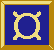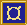# ¤ postProcessHigh, preProcessHigh, et al

## Types

```postProcessHigh :: SP a b -> F c a -> F c b
preProcessHigh :: F a b -> SP c a -> F c b
postProcessLow :: SP TCommand TCommand -> F a b -> F a b
preProcessLow :: F a b -> SP TEvent TEvent -> F a b
postMapHigh :: (a -> b) -> F c a -> F c b
preMapHigh :: F a b -> (c -> a) -> F c b
preMapLow :: F a b -> (TEvent -> TEvent) -> F a b
postMapLow :: (TCommand -> TCommand) -> F a b -> F a b
prepostMapHigh :: (a -> b) -> (c -> d) -> F b c -> F a d
prepostMapLow :: (a -> b) -> (c -> d) -> Fa b c e f -> Fa a d e f
>^^=< :: SP a b -> F c a -> F c b
infixr 7 >^^=<
>=^^< :: F a b -> SP c a -> F c b
infixl 6 >=^^<
>..=< :: SP TCommand TCommand -> F a b -> F a b
infixr 5 >..=<
>=..< :: F a b -> SP TEvent TEvent -> F a b
infixl 6 >=..<
>^=< :: (a -> b) -> F c a -> F c b
infixr 7 >^=<
>=^< :: F a b -> (c -> a) -> F c b
infixl 6 >=^<
>.=< :: (TCommand -> TCommand) -> F a b -> F a b
infixr 6 >.=<
>=.< :: F a b -> (TEvent -> TEvent) -> F a b
infixl 6 >=.<
```

## Description

These functions are used to attach pre- and post-processors to fudgets.

There are two sets of operators. As suggested by the type signatures, the ones with `Map` (or a single `^` or `.`) as part of their names take functions that operate on single stream elements.

The operators with `Process` (or double `^^` or `..`) as part of their names take stream processors as arguments.

## Example

` (*2) >^=< fud   -- doubles the (numeric) output from fud`

## Equalities

The following equalities hold (except that the tagging of low level messages will be different). The left hand sides are likely to be more efficient.

```  sp  >^^=< fud  =  absF sp >==<  fud
fud >=^^< sp   =  fud     >==<  absF sp
f   >^=<  fud  =  mapSP f >^^=< fud
fud >=^<  f    =  fud     >=^^< mapSP f
```